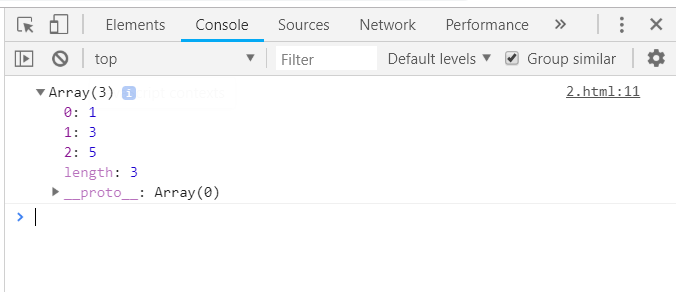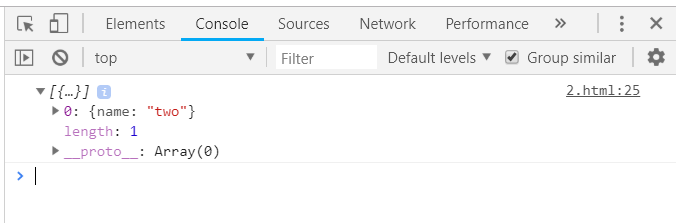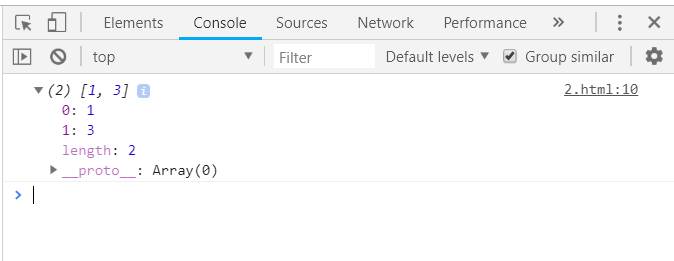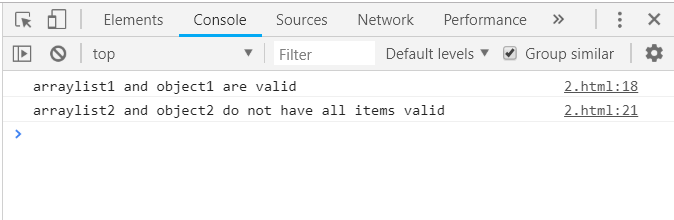Open in App
Not now

# Underscore.js _.reject Function

• Last Updated : 24 Nov, 2021

The Underscore.js is a JavaScript library that provides a lot of useful functions that helps in the programming in a big way like the map, filter, invoke etc even without using any built-in objects.
The _.reject() function is used to give the answer which does not match with the given condition. It is used to find out those elements from the list which does not satisfy the given condition and then to make changes to them only. When all the elements of the list are passed to the function/iterates and no more elements remains then the _.reject() loop ends. It is opposite of _.filter() function as filter selects those items which satisfy the given condition.

Syntax:

`_.reject( list, predicate, [context] )`

Parameters: This function accepts three parameters as mentioned above and described below:

• List: This parameter contains the element list.
• Predicate: This parameter contains the condition which is used to reject the elements.
• Context: It is the text which is used to display. It is optional parameter.

Return values: This function returns the array of elements which does not fulfill the condition of _.reject() function.

Passing a list of numbers to _.reject() function: The ._reject() function takes the element from the list one by one and do the operations on the code. Below is the example to find the odd elements from the list.

Example:

 `<``html``> ` `    ``<``head``> ` `        ``<``script` `type``=``"text/javascript"` `src` `=  ` `        ``"https://cdnjs.cloudflare.com/ajax/libs/underscore.js/1.9.1/underscore-min.js"` `> ` `        `` ` `        ``<``script` `type``=``"text/javascript"` `         ``src``=``"https://cdnjs.cloudflare.com/ajax/libs/underscore.js/1.9.1/underscore.js"``> ` `         `` ` `    `` ` `       `  `    ``<``body``> ` `        ``<``script` `type``=``"text/javascript"``> ` `              ``var odds = _.reject([1, 2, 3, 4, 5, 6],  ` `                           ``function(num){ return num % 2 == 0; }); ` `              ``console.log(odds);  ` `        `` ` `    ``  ` ` `

Output:Passing a structure to the _.reject() function: Choose one property of the items on the basis of which the condition will be applied. Below used the ‘disabled’ property. Since the ‘item2’ doesn’t have the disabled property so it is rejected and given as output.

Example:

 `<``html``>  ` `    ``<``head``> ` `        ``<``script` `type``=``"text/javascript"` `src` `=  ` `        ``"https://cdnjs.cloudflare.com/ajax/libs/underscore.js/1.9.1/underscore-min.js"``> ` `        `` ` `        ``<``script` `type``=``"text/javascript"` `         ``src``=``"https://cdnjs.cloudflare.com/ajax/libs/underscore.js/1.9.1/underscore.js"``> ` `         `` ` `    ``  ` `    ``<``body``> ` `        ``<``script` `type``=``"text/javascript"``> ` `             ``var data = { ` `                ``stuff: { ` `                    ``item1: { ` `                        ``name: "one", ` `                        ``disabled: true ` `                    ``}, ` `                  ``item2: { ` `                        ``name: "two" ` `                    ``} ` `                ``} ` `            ``}; ` `            ``data.stuff = _.reject(data.stuff, function(val) { ` `                ``return val.disabled; ` `            ``}); ` `        ``console.log(data.stuff); ` `        `` ` `    `` ` ` `

Output:Passing a list of numbers and a value to match with item: The elements from the array which does not match with the given number are rejected and hence displayed as array in output.

Example:

 `<``html``> ` `    ``<``head``> ` `        ``<``script` `type``=``"text/javascript"` `src` `=  ` `        ``"https://cdnjs.cloudflare.com/ajax/libs/underscore.js/1.9.1/underscore-min.js"` `> ` `        `` ` `        ``<``script` `type``=``"text/javascript"` `src` `=  ` `        ``"https://cdnjs.cloudflare.com/ajax/libs/underscore.js/1.9.1/underscore.js"``> ` `    ``       ` `    ``<``body``> ` `        ``<``script` `type``=``"text/javascript"``> ` `             ``console.log( _.reject({one: 1, two: 2, three: 3}, function(val, key){ ` `                  ``return val === 2; ` `             ``})); ` `        `` ` `    ``   ` ` `

Output:Using two _.reject() function together: Pass different objects to each _.reject() function and then use the following results together by using the logical operators like &&, ||, ! etc. Here, both the 2 objects and the 2 arraylists are rejected as they does not fulfill the given condition so they are given as output.

Example:

 `<``html``>   ` `    ``<``head``> ` `        ``<``script` `type``=``"text/javascript"` `src` `=  ` `        ``"https://cdnjs.cloudflare.com/ajax/libs/underscore.js/1.9.1/underscore-min.js"` `> ` `        `` ` `        ``<``script` `type``=``"text/javascript"` `src` `=  ` `        ``"https://cdnjs.cloudflare.com/ajax/libs/underscore.js/1.9.1/underscore.js"``> ` `        `` ` `    ``   ` `    ``<``body``> ` `        ``<``script` `type``=``"text/javascript"``> ` `             ``var arraylist1 = [true]; ` `             ``var arraylist2 = [null, {} , undefined, {}]; ` `             ``var object1 = {prop1: true}; ` `             ``var object2 = { ` `                    ``prop1: null, ` `                    ``prop2: true,prop3: true, ` `             ``};  ` `             ``if (_.reject(arraylist1) && _.reject(object1)) { ` `                    ``console.log('arraylist1 and object1 are valid'); ` `             ``} ` `             ``if (_.reject(arraylist2) && _.reject(object2)) { ` `                    ``console.log('arraylist2 and object2 do not have all items valid'); ` `             ``} ` `        `` ` `    ``   ` ` `

Output:My Personal Notes arrow_drop_up
Related Articles